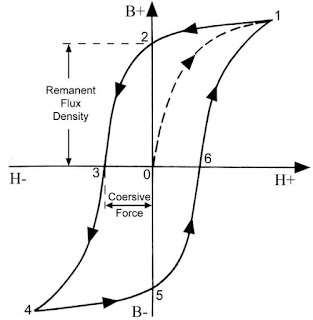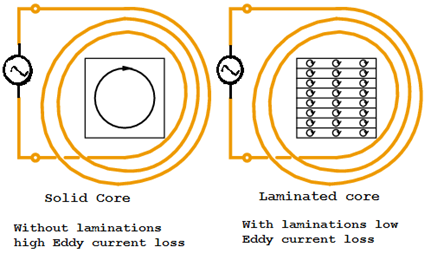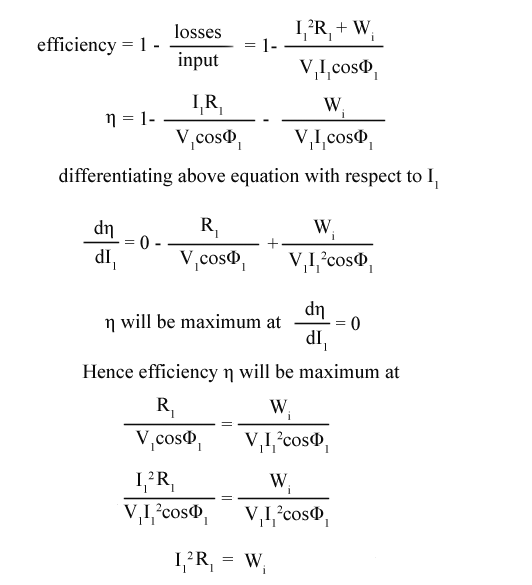# Losses In Transformers ; Hysteresis Loss, Eddy Current Loss, Efficiency

0
564

### Losses In Transformers ; Hysteresis Loss, Eddy Current Loss, Efficiency

#### We have discussed electrical transformer construction, working When it comes to efficiency of the transformer it is above 95%.In modern power transformers, we can get above 98% of efficiency. An electrical transformer is a static device, so no rotating losses or frictional, windage losses.So no mechanical losses occur in transformer.We consider only electrical losses in the transformer.Now read efficiency of transformer and losses in transformer below.### (i) Core Losses Or Iron Losses in Transformer

####The hysteresis loss in transformer depends on the volume and grade of the iron, frequency of magnetic reversals and value of flux density. It can be given by, Steinmetz formula:

Wh= ηBmax1.6fV (watts)

where,   η = Steinmetz hysteresis constant
V = volume of the core in m3

#### How to reduce Eddy current loss in transformer?### Efficiency Of Transformer

#### Iron loss = Wi